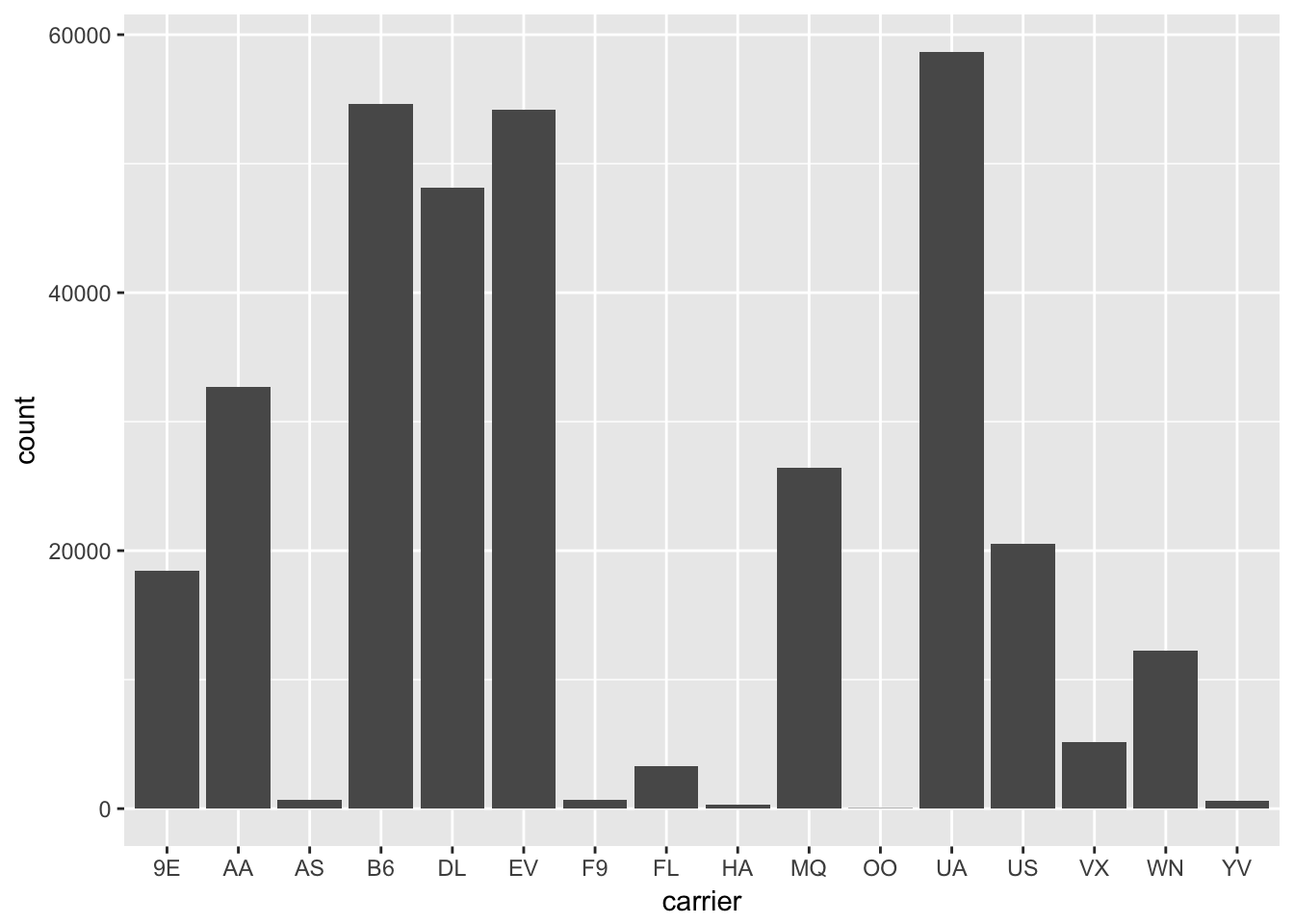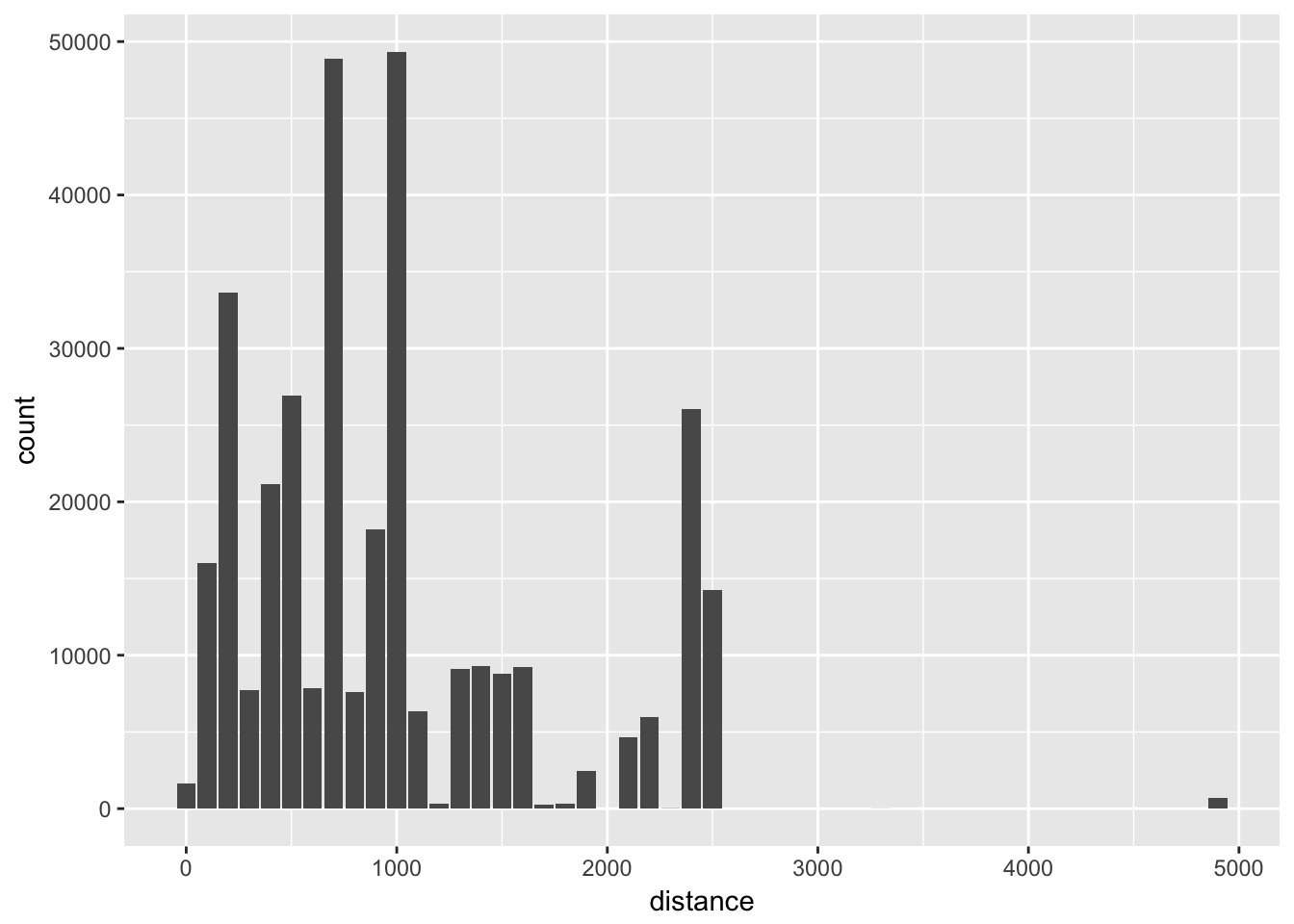# Chapter 6 Calculation

MongoDB has two methods for performing in-database calculations: aggregation pipelines and mapreduce. The aggregation pipeline system provides better performance and more coherent interface. However, map-reduce operations provide some flexibility that is not presently available in the aggregation pipeline.

## 6.1 Aggregate

The `aggregate`() method allows you to run an aggregation pipeline. For example the pipeline below calculates the total flights per carrier and the average distance:

``````stats <- flt\$aggregate(
'[{"\$group":{"_id":"\$carrier", "count": {"\$sum":1}, "average":{"\$avg":"\$distance"}}}]',
options = '{"allowDiskUse":true}'
)
names(stats) <- c("carrier", "count", "average")
print(stats)``````
``````#>    carrier count   average
#> 1       OO    32  500.8125
#> 2       F9   685 1620.0000
#> 3       YV   601  375.0333
#> 4       EV 54173  562.9917
#> 5       FL  3260  664.8294
#> 6       9E 18460  530.2358
#> 7       AS   714 2402.0000
#> 8       US 20536  553.4563
#> 9       MQ 26397  569.5327
#> 10      UA 58665 1529.1149
#> 11      DL 48110 1236.9012
#> 12      B6 54635 1068.6215
#> 13      VX  5162 2499.4822
#> 14      WN 12275  996.2691
#> 15      HA   342 4983.0000
#> 16      AA 32729 1340.2360``````

Let’s make a pretty plot:

``````library(ggplot2)
ggplot(aes(carrier, count), data = stats) + geom_col()``````Check the MongoDB manual for detailed description of the pipeline syntax and supported options.

## 6.2 Aggregation Iterators

If you are expecting a lot of data or do not want to automatically simplify the results into data frames, run the aggregation with `iterate = TRUE`:

``````iter <- flt\$aggregate(
'[{"\$group":{"_id":"\$carrier", "count": {"\$sum":1}, "average":{"\$avg":"\$distance"}}}]',
options = '{"allowDiskUse":true}',
iterate = TRUE
)``````

This will return the results in the form of an iterator just like when using the iterate method. You can then iterate over the results one by one or in pages:

``````# Get the first 10 results
iter\$json(10)``````
``````#>   "{ \"_id\" : \"OO\", \"count\" : 32, \"average\" : 500.8125 }"
#>   "{ \"_id\" : \"F9\", \"count\" : 685, \"average\" : 1620.0 }"
#>   "{ \"_id\" : \"YV\", \"count\" : 601, \"average\" : 375.033277870216 }"
#>   "{ \"_id\" : \"EV\", \"count\" : 54173, \"average\" : 562.9917301977 }"
#>   "{ \"_id\" : \"FL\", \"count\" : 3260, \"average\" : 664.829447852761 }"
#>   "{ \"_id\" : \"9E\", \"count\" : 18460, \"average\" : 530.235752979415 }"
#>   "{ \"_id\" : \"AS\", \"count\" : 714, \"average\" : 2402.0 }"
#>   "{ \"_id\" : \"US\", \"count\" : 20536, \"average\" : 553.456271912739 }"
#>   "{ \"_id\" : \"MQ\", \"count\" : 26397, \"average\" : 569.532712050612 }"
#>  "{ \"_id\" : \"UA\", \"count\" : 58665, \"average\" : 1529.11487258161 }"``````

## 6.3 Map/Reduce

The `mapreduce()` method allow for running a custom in-database mapreduce job by providing custom `map` and `reduce` JavaScript functions. Running JavaScript is slower using the aggregate system, but you can implement fully customized database operations.

Below is a simple example to do “binning” of distances to create a histogram.

``````# Map-reduce (binning)
histdata <- flt\$mapreduce(
map = "function(){emit(Math.floor(this.distance/100)*100, 1)}",
reduce = "function(id, counts){return Array.sum(counts)}"
)
names(histdata) <- c("distance", "count")
print(histdata)``````
``````#>    distance count
#> 1         0  1633
#> 2       100 16017
#> 3       200 33637
#> 4       300  7748
#> 5       400 21182
#> 6       500 26925
#> 7       600  7846
#> 8       700 48904
#> 9       800  7574
#> 10      900 18205
#> 11     1000 49327
#> 12     1100  6336
#> 13     1200   332
#> 14     1300  9084
#> 15     1400  9313
#> 16     1500  8773
#> 17     1600  9220
#> 18     1700   243
#> 19     1800   315
#> 20     1900  2467
#> 21     2100  4656
#> 22     2200  5997
#> 23     2300    19
#> 24     2400 26052
#> 25     2500 14256
#> 26     3300     8
#> 27     4900   707``````

From this data we can create a pretty histogram:

``````library(ggplot2)
ggplot(aes(distance, count), data = histdata) + geom_col()``````Obviously we could have done binning in R instead, however if we are dealing with loads of data, doing it in database can be much more efficient.